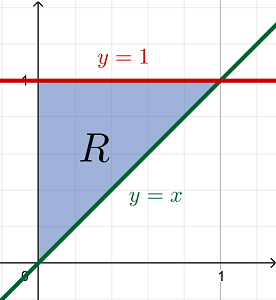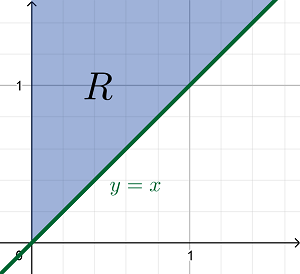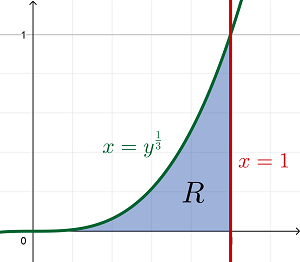# Sketch the region of integration, reverse the order of integration and then evaluate the...

## Question:

Sketch the region of integration, reverse the order of integration and then evaluate the following integrals.

{eq}a) \ \ \int^1_0 \int^1_x e^{-y^2}dy\ dx \\ b) \ \ \int^\infty_0 \int^\infty_x \frac {e^{-y}}{y}dy \ dx\\ c) \ \ \int^1_0 \int^1_{y^\frac {1}{3}} \frac {1}{\sqrt{1+x^2}}dx \ dy {/eq}

## Double Integrals:

Consider the function {eq}F(x,y) {/eq} and the region {eq}D {/eq} on the {eq}xy {/eq}-plane. The integral of this given function over the said planar region is expressed as the double integrals:

$$\iint \limits_R F(x,y) \,dA$$

Type-I Region {eq}R {/eq}

The planar region of integration is a Type I Region when it is defined between two functions of {eq}x {/eq}.

Suppose {eq}R {/eq} is indeed a Type I Region such that on the interval {eq}a \le x \le b {/eq}, the region is bounded above and below by {eq}y = g_2(x) \text{ and } y = g_1(x) {/eq}, respectively.

Then, the double integrals can be expressed as the iterated integrals over a type-I region:

$$\iint \limits_R F(x,y) \,dA = \int_a^b \int_{g_1(x)}^{g_2(x)} F(x,y) \,dy \,dx$$

Type-II Region {eq}R {/eq}

In contrast, the planar region of integration is a Type II Region when it is defined between two functions of {eq}y {/eq}.

Suppose {eq}R {/eq} is instead defined as a Type II Region bounded on the right and on the left by {eq}x = h_2(y) \text{ and } x = h_1(y) {/eq}, respectively, on the interval {eq}c \le y \le d {/eq}.

The double integrals can then be expressed as the iterated integrals over a type-II region:

$$\iint \limits_R F(x,y) \,dA = \int_c^d \int_{h_1(y)}^{h_2(y)} F(x,y) \,dx \,dy$$

Let {eq}R {/eq} be the region of integration of each of the following integrals:

A. {eq}\begin{align*} \int_0^1 \int_x^1 e^{-y^2} \,dy \,dx \end{align*} {/eq}

Based on the order and limits of integration, the region of integration {eq}R {/eq} is defined as a Type I Region bounded above by {eq}y = 1 {/eq} and below by {eq}y = x {/eq} on the interval {eq}0 \le x \le 1 {/eq}.To reverse the order of integration, {eq}R {/eq} must be defined as a Type II Region using functions of {eq}y {/eq}.

Based on the graph, this region of integration is also bounded on the right by {eq}x = y {/eq} and on the left by {eq}x = 0 {/eq} on the interval {eq}0 \le y \le 1 {/eq}.

Therefore, the iterated integrals can be evaluated as with reversed order of integration as:

{eq}\begin{align*} \int_0^1 \int_x^1 e^{-y^2} \,dy \,dx &= \int_0^1 \int_0^y e^{-y^2} \,dx \,dy & \left[ \text{For all constant } k \,,\, \int k \cdot f(x) \,dx = k \int f(x)\,dx \right] \\ &= \int_0^1 e^{-y^2} \int_0^y dx \,dy & \text{[Integrate with respect to } x \text{]} \\ &= \int_0^1 \left. e^{-y^2} \left( x \right) \right|_0^y \,dy & \text{[Fundamental Theorem of Calculus]} \\ &= \int_0^1 e^{-y^2} ( y - 0 ) \,dy \\ &= \int_0^1 ye^{-y^2} \,dy & \text{[Substitute } u = - y^2 \text{ such that } du = -2y \,dy \,,\, u(0) = -0^2 = 0 \text{ and } u(1) = -1^2 = -1 ] \\ &= \int_0^{-1} - \frac{1}{2} e^u \,du & \text{[Integrate with respect to } u \text{]} \\ &= \left. - \frac{1}{2} e^u \right|_0^{-1} & \text{[Fundamental Theorem of Calculus]} \\ &= - \frac{1}{2} (e^{-1} - e^0) \\ &= - \frac{1}{2} \left( \frac{1}{e} - \frac{e}{e} \right) \\ \int_0^1 \int_x^1 e^{-y^2} \,dy \,dx &= \boxed{ \frac{e - 1}{2e} } \end{align*} {/eq}

B. {eq}\begin{align*} \int_0^\infty \int_x^\infty \frac{ e^{-y} }{y} \,dy \,dx \end{align*} {/eq}

Based on the order and limits of integration, the region of integration {eq}R {/eq} is defined as another Type I Region which lies above the line {eq}y = x {/eq} on the interval {eq}0 \le x \le \infty {/eq}.Similar to Part A, the region {eq}R {/eq} must be defined as a Type II Region using functions of {eq}y {/eq}.

Based on the graph, it can be defined in terms of {eq}y {/eq} using the inequalities {eq}0 \le y \le \infty \text{ and } 0 \le x \le y {/eq}. Therefore, the given iterated integrals can be evaluated as:

{eq}\begin{align*} \int_0^\infty \int_x^\infty \frac{ e^{-y} }{y} \,dy \,dx &= \int_0^\infty \int_0^y \frac{ e^{-y} }{y} \,dx \,dy & \left[ \text{For all constant } k \,,\, \int k \cdot f(x) \,dx = k \int f(x)\,dx \right] \\ &= \int_0^\infty \frac{ e^{-y} }{y} \int_0^y dx \,dy & \text{[Integrate with respect to } x \text{]} \\ &= \int_0^\infty \left. \frac{ e^{-y} }{y} \left( x \right) \right|_0^y \,dy & \text{[Fundamental Theorem of Calculus]} \\ &= \int_0^\infty \frac{ e^{-y} }{y} (y - 0) \,dy \\ \int_0^\infty \int_x^\infty \frac{ e^{-y} }{y} \,dy \,dx &= \int_0^\infty e^{-y} \,dy & \text{[Since the integral is improper, first evaluate its indefinite integral]} \\ \\ \int e^{-y} \,dy &= \int - e^u \,du & \text{[Let } u = -y \text{ and } du = - dy \text{. Integrate with respect to } u \text{]} \\ &= - e^u + C & \text{[Substitute back to } u = - y ] \\ \int e^{-y} \,dy &= - \frac{1}{e^y} + C & \text{[Evaluate at the limits of integration]} \\ \\ \int_0^\infty \int_x^\infty \frac{ e^{-y} }{y} \,dy \,dx &= \int_0^\infty e^{-y} \,dy \\ &= - \frac{1}{e^\infty} + \frac{1}{e^0} & \left[ \text{Note that when } b > 1 \,,\, \frac{1}{b^\infty} \rightarrow 0 \right] \\ &= 0 + \frac{1}{1} \\ \int_0^\infty \int_x^\infty \frac{ e^{-y} }{y} \,dy \,dx &= \boxed{ 1 } \end{align*} {/eq}

C. {eq}\begin{align*} \int_0^1 \int_{y^\frac{1}{3}}^1 \frac{1}{\sqrt{1 + x^2}} \,dx \,dy \end{align*} {/eq}

Based on the order and limits of integration, the region of integration {eq}R {/eq} is defined as a Type II Region bounded on the left by {eq}x = y^\frac{1}{3} {/eq} and on the right by {eq}x = 1 {/eq} on the interval {eq}0 \le y \le 1 {/eq}.Since {eq}x = y^\frac{1}{3} {/eq} can also be expressed as {eq}y = x^3 {/eq}, reversing the order of integration, the region {eq}R {/eq} is defined as a Type I Region using the inequalities {eq}0 \le x \le 1 \text{ and } 0 \le y \le x^3 {/eq}.

Therefore, the given iterated integrals can be evaluated as:

{eq}\begin{align*} \int_0^1 \int_{y^\frac{1}{3}}^1 \frac{1}{\sqrt{1 + x^2}} \,dx \,dy &= \int_0^1 \int_0^{x^3} \frac{1}{\sqrt{1 + x^2}} \,dy \,dx & \left[ \text{For all constant } k \,,\, \int k \cdot f(x) \,dx = k \int f(x)\,dx \right] \\ &= \int_0^1 \frac{1}{\sqrt{1 + x^2}} \int_0^{x^3} dy \,dx & \text{[Integrate with respect to } y \text{]} \\ &= \int_0^1 \left. \frac{1}{\sqrt{1 + x^2}} \left( y \right) \right|_0^{x^3} \,dx & \text{[Fundamental Theorem of Calculus]} \\ &= \int_0^1 \frac{1}{\sqrt{1 + x^2}} (x^3 - 0) \,dx \\ &= \int_0^1 \frac{x^3}{\sqrt{1 + x^2}} \,dx & \left[ \text{Let } u = \sqrt{1 + x^2} = (1 + x^2)^\frac{1}{2} \text{ such that } x^2 = u^2 - 1 \text{ and } du = \frac{x\,dx}{\sqrt{1 + x^2}} \right] \\ &= \int_{u(0)}^{u(1)} (u^2 - 1) \,du & \left[ \text{The new limits of integration are } u(0) = \sqrt{1 + 0^2} = 1 \text{ and } u(1) = \sqrt{1 + 1^2} = \sqrt{2} \right] \\ &= \int_1^\sqrt{2} (u^2 - 1) \,du & \text{[Integrate with respect to } u \text{]} \\ &= \left. \left( \frac{u^3}{3} - u \right) \right|_1^\sqrt{2} & \text{[Fundamental Theorem of Calculus]} \\ &= \left( \frac{\sqrt{2}^3}{3} - \sqrt{2} \right) - \left( \frac{1^3}{3} - 1 \right) \\ &= - \frac{\sqrt{2}}{3} + \frac{2}{3} \\ \int_0^1 \int_{y^\frac{1}{3}}^1 \frac{1}{\sqrt{1 + x^2}} \,dx \,dy &= \boxed{ \frac{2 - \sqrt{2}}{3} } \end{align*} {/eq}